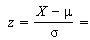# Standardized Variables: Definition, Examples

Share on

Statistics Definitions > Standardized Variables

## What are Standardized Variables?

A standardized variable means something slightly different according to where you’re using it.

1. Use in Probability and Statistics.
2. Use in General Science (including biology).

## 1. Use in Probability and Statistics

In statistics, standardized variables are variables that have been standardized to have a mean of 0 and a standard deviation of 1. The variables are rescaled using the z-score formula. Standardizing makes it easier to compare scores, even if those scores were measured on different scales. It also makes it easier to read results from regression analysis and ensures that all variables contribute to a scale when added together.

To standardize a variable, use the following formula:1. Subtract the mean, μ, from the value you want to convert, X.
2. Divide the result from Step 1 by the standard deviation, σ.

For more information on the process, including a step by step video, see: how to calculate a z-score.

## 1. In General Science.

Sometimes, authors and teachers will use the term “standardized variables” as another name for control variables — the variables you keep constant in an experiment. When you keep these variables constant you can figure out if your independent variable is having an effect. For example, you might have an experiment to see if plants grow better with tap water or spring water. The variables you would need to standardize include: light, fertilizer and soil quality.

Calling control variables “standardized” is an informal practice that can quickly become confusing when you try to interpret the results from experiments, because you’ll often need to turn results in standardized variables with a z-score formula (as outlined in the first section above). If you already refer to controls as standardized, and then you want to convert results to standardized scores, you could end up trying to standardize variables that are already standardized. To avoid this mind-bending confusion, I recommend that you call the variables you need to hold constant “control variables.”

Reference:
Helms, D et. al. Biology in the Laboratory. Retrieved July 21 2016 from here.

CITE THIS AS:
Stephanie Glen. "Standardized Variables: Definition, Examples" From StatisticsHowTo.com: Elementary Statistics for the rest of us! https://www.statisticshowto.com/standardized-variables/
---------------------------------------------------------------------------Need help with a homework or test question? With Chegg Study, you can get step-by-step solutions to your questions from an expert in the field. Your first 30 minutes with a Chegg tutor is free!